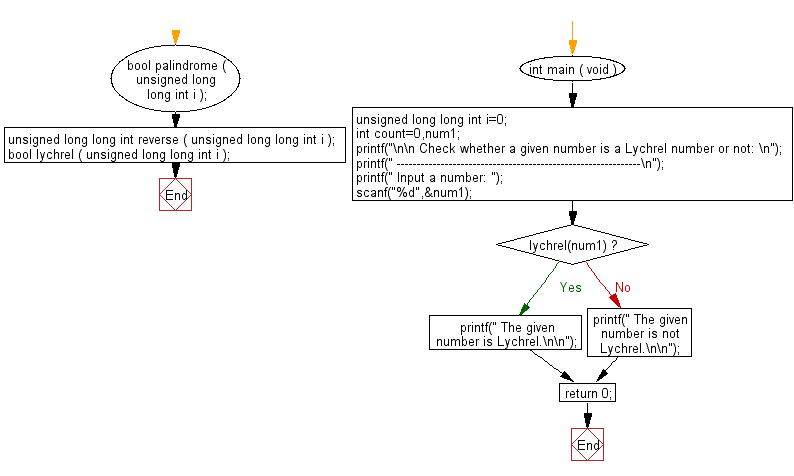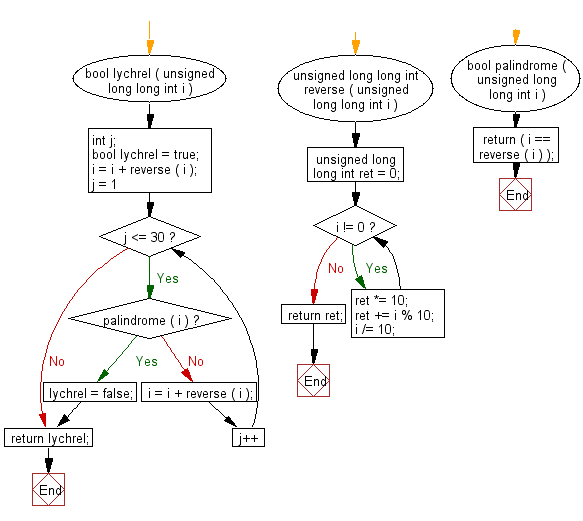﻿ C Program: Check whether a number is Lychrel number or not - w3resource# C Exercises: Check whether a number is Lychrel number or not

## C Numbers: Exercise-8 with Solution

Write a program in C to check whether a number is Lychrel number or not.

Test Data
Input a number: 196

Sample Solution:

C Code:

``````# include <stdio.h>
# include <stdbool.h>
# include <stdlib.h>

bool palindrome ( unsigned long long int i );
unsigned long long int reverse ( unsigned long long int i );
bool lychrel ( unsigned long long int i );

int main ( void )
{
unsigned long long int i=0;
int count=0,num1;
printf("\n\n Check whether a given number is a Lychrel number or not: \n");
printf(" -------------------------------------------------------------\n");
printf(" Input a number: ");
scanf("%d",&num1);
if(lychrel(num1))
{
printf(" The given number is Lychrel.\n\n");
}
else
{
printf(" The given number is not Lychrel.\n\n");
}
return 0;
}
bool lychrel ( unsigned long long int i )
{
int j; /*iteration counter*/
bool lychrel = true;
i = i + reverse ( i );

for ( j = 1; j <= 30 ; j++ )
{
if ( palindrome ( i ) )
{
lychrel = false;
break;
}
i = i + reverse ( i );
}

return lychrel;
}
unsigned long long int reverse ( unsigned long long int i )
{
unsigned long long int ret = 0;
while ( i != 0 )
{
ret *= 10;
ret += i % 10;
i /= 10;
}
return ret;
}

bool palindrome ( unsigned long long int i )
{
return ( i == reverse ( i ) );
}
```
```

Sample Output:

``` Input a number: 196
The given number is Lychrel.
```

Pictorial Presentation:Flowchart:C Programming Code Editor:

What is the difficulty level of this exercise?

Test your Programming skills with w3resource's quiz.

﻿

## C Programming: Tips of the Day

Why do C and C++ compilers allow array lengths in function signatures when they're never enforced?

It is a quirk of the syntax for passing arrays to functions.

Actually it is not possible to pass an array in C. If you write syntax that looks like it should pass the array, what actually happens is that a pointer to the first element of the array is passed instead.

Since the pointer does not include any length information, the contents of your [] in the function formal parameter list are actually ignored.

Ref : https://bit.ly/3fhlvdH